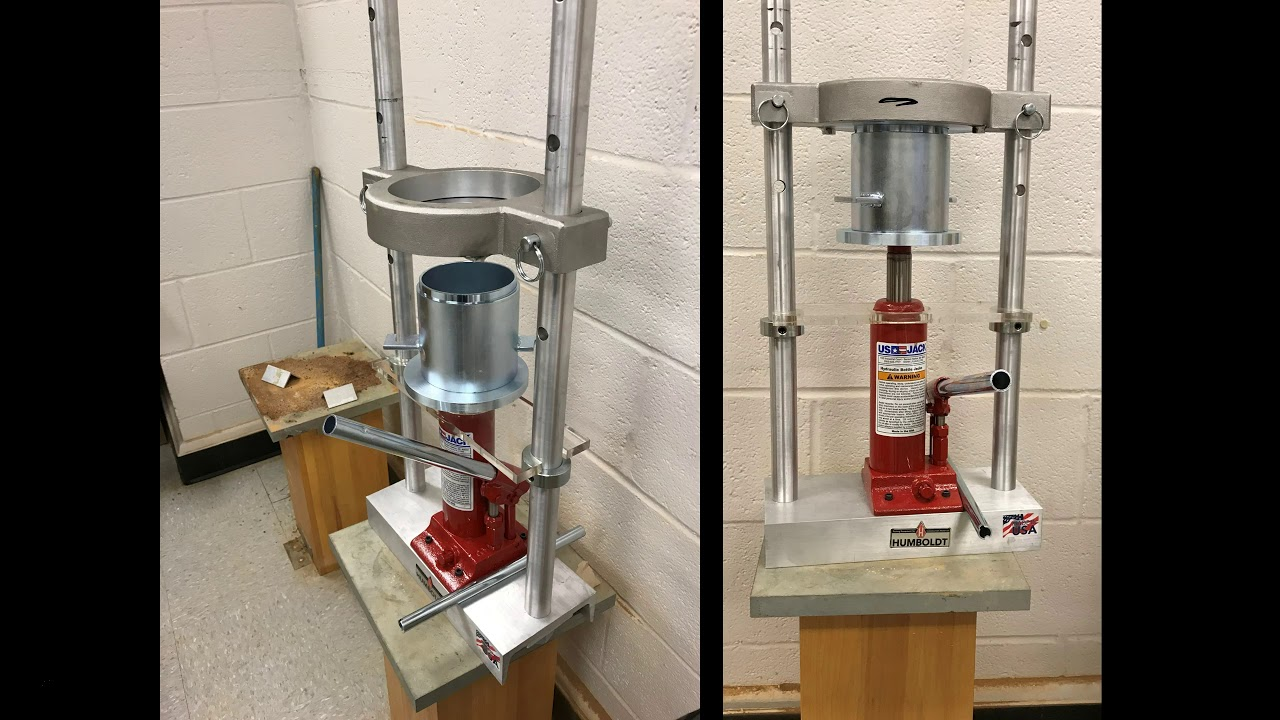# How to Test Compaction of Soil?

14 December 2018 Off

# How to Test Compaction of Soil?

Compaction test is conducted in the laboratory to determine the relation between the dry density and the water content of the given soil compacted with standard compaction energy and to determine the OMC corresponding to the MDD.

The OMC obtained from the laboratory compaction test will help in deciding the amount of water to be used for compaction in the field. The MDD obtained from the laboratory compaction test helps in knowing the dry density achievable in the field compaction and also as a check for quality control.

Based on the compacted energy used in compacting the soil in the laboratory test, the laboratory compaction tests are of two types:

1. Standard Proctor test.

2. Modified Proctor test.

#### 1. Standard Proctor Test:In this test, the soil is compacted in three layers, each layer subjected to blows of a rammer with falling weight of 5.5 pounds (2.6 kgf) falling through a height of 12 in. in a cylindrical mold of internal diameter of 4 in. and effective height of 4.6 in.

The compaction parameters in a standard Proctor test are – W is the weight of rammer blow = 5.5 pounds, h is the height of fall = 12 in. = 1 ft, n is the number of blows per layer = 25, l is the number of layers = 3, and Vm is the volume of the mold = 1/30 cubic ft. The total compaction energy imparted on the soil per unit volume in this test is –IS – 2720 (Part 7) – 1980 recommends the Indian Standard light compaction method based on standard Proctor test in metric system. The standard Proctor test or IS light compaction can be used as a criteria for the compaction of subgrades on highways and earth dams, where light rollers are used.

#### 2. Modified Proctor Test:Advances in construction technology resulted in the development of heavier rollers, which impart higher compaction energy during field compaction. To provide a laboratory control criterion for the higher compaction energy, the modified Proctor test was developed and standardized by the American Association of State Highway (and Transportation) Officials (AASHO or AASHTO) and by US Army Corps of Engineers for airfield construction.

In modified Proctor test, the soil is compacted in the same mold as in standard Proctor test, which has internal diameter of 4 in. and affective height of 4.6 in. giving a total internal volume of 57.805 cubic in. or 1/30 cubic ft. The rammer is bigger with a hammer of weight 10 pounds (4.5 kgf) falling through a height of 18 in. The soil is compacted in five layers, each layer being given 25 blows.

The compaction parameters in modified Proctor test are as follows – W is the weight of hammer blow = 10 pounds, h is the height of fall = 18 in. = 1.5 ft, n is the number of blows per layer = 25,l is the number of layers = 5, and Vm is the volume of the mold = 1/30 cubic ft.

The compaction energy imparted to the soil, per unit volume, in the modified Proctor test is:

Thus, the compaction energy in the modified Proctor test is (56250/12375) 4.55 times that in standard Proctor test.

If the soil fraction retained on 20 mm sieve is more than 5%, a bigger mold of 5.9 in. (15 cm) internal diameter and 5 in. (12.73 cm) internal height giving a total volume of 137.04 cubic in. or 1/12.611 cubic ft (2250 cm3) is used. When the bigger mold is used, the soil is compacted with 56 blows for each layer.

IS 2720 (Part VII) 1980 and Part VIII-1983 have recommended procedures corresponding to these two tests as follows:

1. IS light compaction test.

2. IS heavy compaction test.

#### 1. IS Light Compaction Test:

The objective of the IS light compaction test is to determine the relation between the water content and the dry density of compacted soil and to determine the MDD and OMC from this test. The compaction energy used to compact the soil corresponds to that of standard Proctor test.

The compaction parameters in IS light compaction test are as follows – W is the weight of hammer blow = 2.6 kgf, h is the height of fall = 31 cm, n is the number of blows per layer = 25, and I is the number of layers = 3, and Vm is the volume of the mold = 1000 cubic centimeter (cc). The total compaction energy imparted on the soil in this test is –

E = Whnl = 2.6 × 31 × 25 × 3 = 6045 kgf cm

The total compaction energy imparted on the soil per unit volume in this test is –

E = 6045/1000 = 6.045 kgf cm/cm3

#### 2. IS Heavy Compaction Test:

The IS heavy compaction test is similar to IS light compaction text except for the following differences:

1. A heavy rammer of 4.9 kgf falling weight that falls through a height of 45 cm is used for compacting the soil in IS heavy compaction.

2. The soil is compacted in five layers of equal thickness in the compaction mold.

3. The initial water content to be used in the first trial is 3%-5% for sandy and gravelly soils and 12%-16% below plastic limit for cohesive soils.

To increase the accuracy of the test results, it is desirable to reduce the increment of water in the region of OMC.